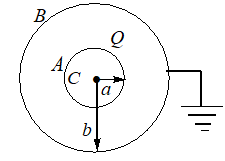A conducting sphere A of radius ‘a’  with charge Q, is placed concentrically inside a conducting shell  B of radius ‘b’. The sphere B is earthed. ‘C’  is the common center of  A and B. Study the following statements.i. The potential at a distance r  from  C, where r

# A conducting sphere A of radius ‘a’  with charge Q, is placed concentrically inside a conducting shell  B of radius ‘b’. The sphere B is earthed. ‘C’  is the common center of  A and B. Study the following statements.i. The potential at a distance r  from  C, where r<a is zero.ii. The Potential difference between  A and  B is $\frac{1}{4\text{π}{\epsilon }_{0}}Q\left(\frac{1}{a}-\frac{1}{b}\right)$iii. The potential at a distance r from C, where  Which of the above statements are correct?

### Solution:

Charge +Q will flow into earth.

Potential at B is zero.Register to Get Free Mock Test and Study Material

+91

Verify OTP Code (required)